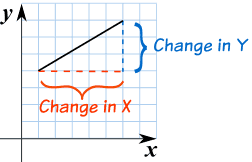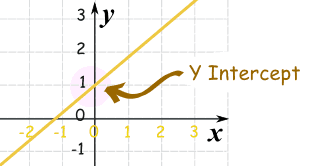# Unit 4: Slope and Y-Interecept

Standard

### Slope Intercept Form:      y = mx + b

The variable m is the slope, it explains the steepness of a line of how slanted the line is.

The variable b is the y-intercept, this is where the line crosses or intersects the y-axis.

https://www.desmos.com/calculator

http://www.shmoop.com/video/slope-intercept-form/

# Slope: m

Slope is how steep a straight line is.
When finding the slope from a line on a graph we use the method of rise over run .
• Rise is how far up
• Run is how far alongYou can also think of it as the change in y over the change in x.EXAMPLE #1:The slope here is 4/6 which can simplify to 2/3.
EXAMPLE #2:In this example the slope is 3/5.

# Practice Problems

TEST YOUR SELF ON LINES HERE!!!!

https://my.hrw.com/wwtb/api/viewer.pl

# Y Intercept: b

Y intercept is where a straight line crosses the Y axis of a graph.

### Example:In the above diagram the line crosses the Y axis at 1.

So the Y intercept is equal to 1.

# Slope Intercept Form

### y = mx + b

m = Slope (how steep the line is)

b = the Y Intercept (where the line crosses the Y axis)\### How do you find “m” and “b”?

• b is easy: just see where the line crosses the Y axis.
• m (the Slope) needs some calculation. Remember to write slope as a fraction.

## Example 1)The fastest and easiest thing to find first when looking at a graph is the y-intercept (b).

Here we see that the line crosses the y-axis at positive 1.

So, b = 1 .

Now to find the slope (m) we will use rise over run:

• Rise is how far up
• Run is how far along

In this example the rise is 2 and the run is 1.

So, m = 2/1 .

Now that I know m = 2/1 and b = 1 I can plug them into the equation for slope intercept form y = mx + b.

y = (2/1) x + 1

^^^^^ This is the equation of the line.